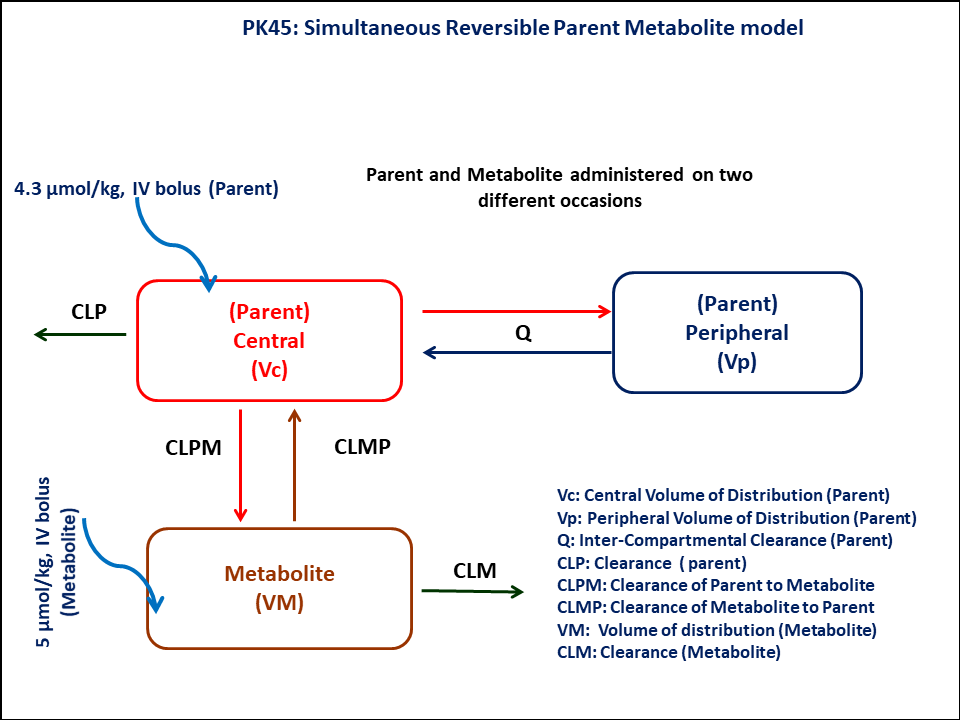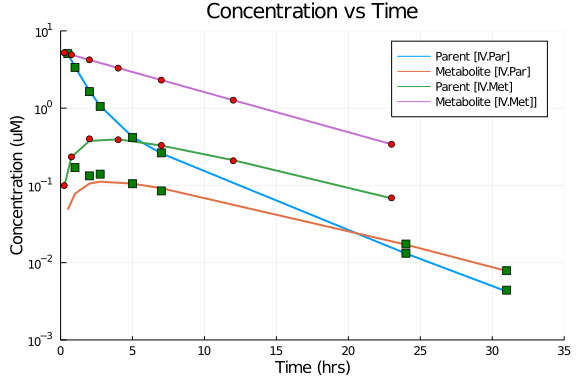# Exercise PK45 - Reversible metabolism of drug A & its metabolite B

### Background

• Structural model - Two compartment model for parent and one compartment Model for metabolite with reversible metabolism

• Route of administration - Administration of parent drug and metabolite on two different occasions

• Dosage Regimen - 4.3 Micromol/Kg of parent & 5 Micromol/Kg of metabolite

• Number of Subjects - 1### Learning Outcome

In this model, you will learn how to build a two compartment parent and one compartment metabolite model with reversible metabolism, while parent and metabolite is admininstered on two different occasions

### Libraries

call the "necessary" libraries to get start.

using Pumas
using Plots
using CSV
using StatsPlots
using Random


### Model

Two compartment model for parent and one compartment model metabolite

pk_45            = @model begin
@param begin
tvvcp        ∈ RealDomain(lower=0)
tvvpp        ∈ RealDomain(lower=0)
tvqp         ∈ RealDomain(lower=0)
tvclp        ∈ RealDomain(lower=0)
tvvcm        ∈ RealDomain(lower=0)
tvclm        ∈ RealDomain(lower=0)
tvclpm       ∈ RealDomain(lower=0)
tvclmp       ∈ RealDomain(lower=0)
Ω            ∈ PDiagDomain(8)
σ²_prop      ∈ RealDomain(lower=0)
end

@random begin
η            ~ MvNormal(Ω)
end

@pre begin
Vcp          = tvvcp * exp(η)
Vpp          = tvvpp * exp(η)
Qp           = tvqp * exp(η)
Clp          = tvclp * exp(η)
Vcm          = tvvcm * exp(η)
Clm          = tvclm* exp(η)
Clpm         = tvclpm * exp(η)
Clmp         = tvclmp * exp(η)
end

@dynamics begin
Centralp'    = (Qp/Vpp)*Peripheralp - (Qp/Vcp)*Centralp - (Clp/Vcp)*Centralp - (Clpm/Vcp)*Centralp + (Clmp/Vcm)*Centralm
Peripheralp' = (Qp/Vcp)*Centralp -  (Qp/Vpp)*Peripheralp
Centralm'    = -(Clm/Vcm)*Centralm - (Clmp/Vcm)*Centralm + (Clpm/Vcp)*Centralp
end

@derived begin
cp           = @. Centralp/Vcp
met          = @. Centralm/Vcm
dv_cp        ~ @. Normal(cp, sqrt(cp^2*σ²_prop))
dv_met       ~ @. Normal(met, sqrt(cp^2*σ²_prop))
end
end

PumasModel
Parameters: tvvcp, tvvpp, tvqp, tvclp, tvvcm, tvclm, tvclpm, tvclmp, Ω, σ
²_prop
Random effects: η
Covariates:
Dynamical variables: Centralp, Peripheralp, Centralm
Derived: cp, met, dv_cp, dv_met
Observed: cp, met, dv_cp, dv_met


### Parameters

Parameters provided for simulation. tv represents the typical value for parameters.

• tvvcp - Volume of distribution of central compartment of Parent (L/kg)

• tvvpp - Volume of distribution of peripheral compartment of Parent (L/kg)

• tvqp - Intercompartmental clearance of Parent (L/hr/kg)

• tvclp - Clearance of Parent (L/hr/kg)

• tvvcm - Volume of distribution of central compartment of Metabolite (L/kg)

• tvclm - Clearance of Metabolite (L/hr/kg)

• tvclpm - Conversion of Parent to Metabolite (L/hr/kg)

• tvclmp - Conversion of Metabolite to Parent (L/hr/kg)

• Ω - Between Subject Variability,

• σ - Residual error

param = (tvvcp   = 0.563,
tvvpp   = 0.424,
tvqp    = 0.115,
tvclp   = 0.343,
tvvcm   = 0.932,
tvclm   = 0.068,
tvclpm  = 0.015,
tvclmp  = 0.046,
Ω       = Diagonal([0.0,0.0,0.0,0.0,0.0,0.0,0.0,0.0]),
σ²_prop = 0.001)

(tvvcp = 0.563, tvvpp = 0.424, tvqp = 0.115, tvclp = 0.343, tvvcm = 0.932,
tvclm = 0.068, tvclpm = 0.015, tvclmp = 0.046, Ω = [0.0 0.0 … 0.0 0.0; 0.0
0.0 … 0.0 0.0; … ; 0.0 0.0 … 0.0 0.0; 0.0 0.0 … 0.0 0.0], σ²_prop = 0.001)


### Dosage Regimen

• A dose of 4.3 μmol/kg of the Parent is administered as a rapid IV Injection over 15 seconds

• A dose of 5 μmol/kg of the metabolite is administered as a rapid IV Injection over 15 seconds

dr_p  = DosageRegimen(4.3,cmt=1,time=0, duration=0.0041)
sub_p = Subject(id=1,events=dr_p)
dr_m  = DosageRegimen(5,cmt=3,time=0,duration=0.0041)
sub_m = Subject(id=2,events=dr_m)
sub   = [sub_p, sub_m]

Population
Subjects: 2
Covariates:


### Simulation

We are going to simulate parent and metabolite concentration profile

Random.seed!(123)
sim_sub = simobs(pk_45,sub,param,obstimes=0:0.001:31)
df1     = DataFrame(sim_sub)


### Dataframe and Plotting

Convert the simulation to a dataframe and use the necessary for plotting

df1_par = filter(x -> x.id == "1", df1)
filter!(x -> x.time in [0.0042,0.0333,0.1667,0.5,1,2,2.75,5,7,24,31], df1_par)
df1_met = filter(x -> x.id == "2", df1)
filter!(x -> x.time in [0.0042,0.0333,0.1333,0.25,0.75,2,4,7,12,23], df1_met)

@df df1_par plot(:time, :cp,  yaxis=:log, linewidth=2, legend = :topright,
label="Parent [IV.Par]", title="Concentration vs Time",
xlabel="Time (hrs)", ylabel="Concentration (uM)",
xlims=(0,35), xticks=[0,5,10,15,20,25,30,35], yticks=[0.001,0.01,0.1,1,10], ylims=(0.001,10))
@df df1_par plot!(:time, :met, label="Metabolite [IV.Par]",linewidth=2)
@df df1_met plot!(:time, :cp, label="Parent [IV.Met]",linewidth=2)
@df df1_met plot!(:time, :met, label="Metabolite [IV.Met]]",linewidth=2)
@df df1_par scatter!(:time, :dv_cp, marker=[:square], label=false, color=:green)
@df df1_par scatter!(:time, :dv_met, marker=[:square], label=false, color=:green)
@df df1_met scatter!(:time, :dv_cp, marker=[:circle], label=false, color=:red)
@df df1_met scatter!(:time, :dv_met, marker=[:circle], label=false, color=:red)